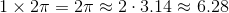# Basic Geometry : How to find the ratio of diameter and circumference

## Example Questions

### Example Question #3 : How To Find The Ratio Of Diameter And Circumference

Letrepresent the area of a circle andrepresent its circumference. Which of the following equations expressesin terms of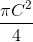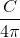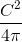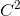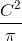Explanation:

The formula for the area of a circle is, and the formula for circumference is. If we solve for C in terms of r, we get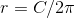.

We can then substitute this value of r into the formula for the area: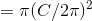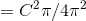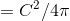### Example Question #371 : Circles

A can of soup has a base area of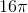. What is the ratio of the can's diameter to its circumference?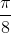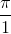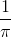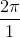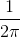Explanation:

If the cirlcle has an area ofthen we need to find a way to determine the diameter of the circle. We must do 4 things:

1. Find the radius of the circle since all we know is the area of the circle.

2. Double the radius to find the diameter.

3. Find the circumference  by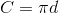4. Write the ratio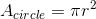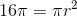Solving to find the radius we get: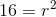So if the radius is 4 then the diameter is: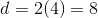Then the circumference is: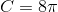The ratio of the diameter to the circumference is: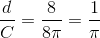### Example Question #2 : How To Find The Ratio Of Diameter And Circumference

The circumference of the base of a silo is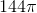ft. What is the ratio of the silo's circumference to its diameter?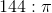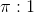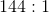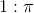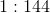Explanation:

Since the silo has base circumference of, then according to the formula for the circumference of a circle: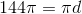Solving for diameter: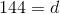So the diameter is 144 ft. Therefore the ratio of the circumference to the diameter would be: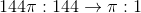### Example Question #3 : How To Find The Ratio Of Diameter And Circumference

The diameter of a certain circle is tripled. Compared to the circumference of the original circle, how many times as large is the circumference of the new circle?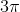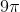Explanation:

The easiest way to find our answer is to try actual values. Imagine we have a circle with a diameter of. Given the formula for circumference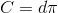our circumference is simply. Tripling the diameter gives a new diameter ofand therefore a new circumference of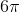. We can then determine the ratio between the two circumferences.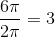Therefore, the new circumference is 3 times as large as the old.

### Example Question #4 : How To Find The Ratio Of Diameter And Circumference

Find the ratio of the diameter to the circumference in a circle with radius r.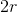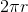Explanation:

To find a ratio, simply divide your two quantities. Remember,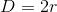Thus,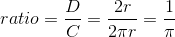### Example Question #5 : How To Find The Ratio Of Diameter And Circumference

What is the circumference to diameter ratio of a circle with a diameter of 15?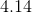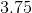Cannot be determined.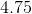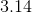Explanation:

First you must find the circumference of a circle with a diameter of 15 with the formula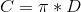.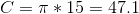Since the ratio of C to D is represented by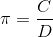, all of the ratios of Circumference to Diameter should approximately equal.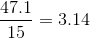### Example Question #6 : How To Find The Ratio Of Diameter And Circumference

In the game of Sumo, both wrestlers are placed on the outer edge of the ring, on opposite sides of each other. If the wrestling ring is a perfect circle and has an area of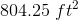, what would be the distance between both wrestlers?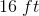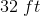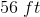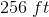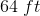Explanation:

With the two wrestlers standing opposite each other on the edge of the ring, the distance between them would constitute the diameter of the circle. We can use the formula for circular area to find the diameter: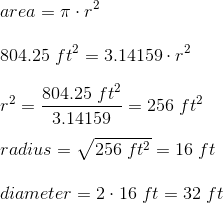### Example Question #7 : How To Find The Ratio Of Diameter And Circumference

True or false: A circle with radius 1 has circumference less than 4.

False

True

The circumference of a circle is equal to its radius multiplied by, so, if the radius of a circle is 1, its circumference is